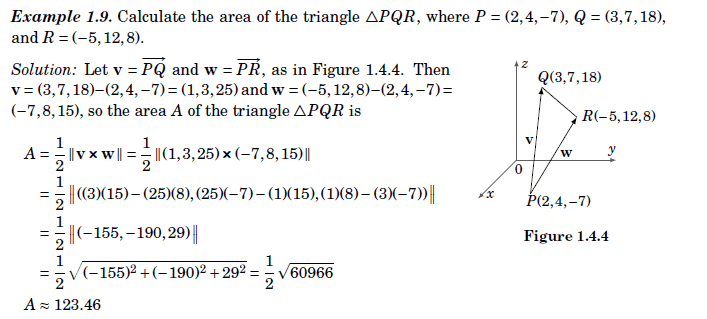# Calculate the area of the triangle- Vector Calculus

• chwala

#### chwala

Gold Member
Homework Statement
see attached.
Relevant Equations
Vector Calculus
##\dfrac {1}{2}####\left\| {v×w}\right\|##
This is the question,Now to my question, supposing the vectors were not given, can we let ##V=\vec {RQ}## and ##W=\vec {RP}##? i tried using this and i was not getting the required area. Thanks...

Last edited by a moderator:
That should give the same answer. Try computing it again.

•WWGD and chwala
You should get the same result.

Last edited:
•chwala
Look up cross product, I think?

•chwala
ok, let me do that again...talk later in the day...I will amend my latex too...laters...

Last edited:
Look up cross product, I think?
I feel silly... The latex rendered slowly.

I already made myself feel silly,but the problem is over specified. You are correct to assume any choice of vector. With only two given, the other is defined, and any choice should give the same answer.

•chwala
Yeah yeah...Great guys same values ##155, 190, -29##...i will post working later. Bingo!

•Yeah yeah...Great guys same values ##155, 190, -29##...i will post working later. Bingo!
A=##\dfrac{1}{2}\|(8,-5,10)×(7,-8,-15)\|=\dfrac{1}{2}(155,190,-29)=\dfrac{1}{2}\sqrt{{155^2+190^2+(-29)^2}}≈123.46##

Last edited:
•A=##\dfrac{1}{2}\|(8,-5,10)×(7,-8,-15)\|=\dfrac{1}{2}(155,190,-29)=\dfrac{1}{2}\sqrt{{155^2+190^2+(-29)^2}}≈123.46##
Nit: The second expression reads as being 1/2 of the vector <155, 190, -29>. What you should have is 1/2 the norm or magnitude of that vector.

•Nit: The second expression reads as being 1/2 of the vector <155, 190, -29>. What you should have is 1/2 the norm or magnitude of that vector.
@Mark44 ...you have keen eyes mate!...I will amend that later in the day...cheers

•i tried using this and i was not getting the required area.

That should give the same answer. Try computing it again.
If I'm working a problem and my result doesn't agree with the published answer, the first thing I do is to make sure I'm working the same problem. If that checks out, I then check my work for errors. Although it's possible that the published answer is wrong, this doesn't happen all that often.

•chwala
A=##\dfrac{1}{2}\|(8,-5,10)×(7,-8,-15)\|=\dfrac{1}{2}\|(155,190,-29)\|=\dfrac{1}{2}\sqrt{{155^2+190^2+(-29)^2}}≈123.46##

Amended post ##9##.

•